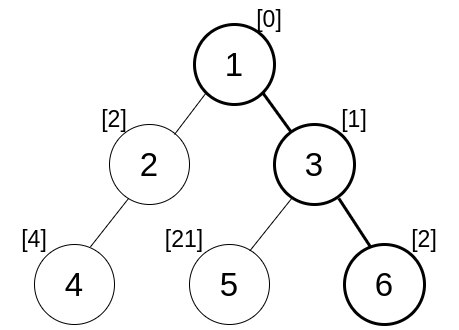# MEXimumaritra741Amra shobai Raja
Limits 1s, 512 MB

Let’s get straight to the point. You are given a tree. In the tree, each node $u$ has a number $a_u$ assigned to it. The score of a node $u$ is defined as the $MEX$ of all the numbers on the simple path from $1$ to $u$.Suppose, $a=[0,2,1,4,21,2]$. The list of values on the path from $1$ to the node $6$ is $[0,1,2]$. The minimum non-negative excluded number or $MEX$ of this list is $3$. So the score of node $6$ is $3$.

You have to print the maximum score among all the nodes.

• A tree is a connected graph with $n$ nodes and $n-1$ edges.

• The MEX of a list of numbers is the minimum non-negative number that is not included in the list. For example, $MEX( [0,1,4,5] )= 2$.

## Input

The first line contains an integer $n ( 1 \leq n \leq 10^5 )$ — the number of nodes.

The second line contains $n$ integers $a_1, a_2, …, a_n$$( 0 \leq a_i \leq 10^{18} )$.

Each of the next $n-1$ lines contains 2 values $u$ and $v$, indicating that there is an edge between $u$ and $v$ in the tree.

## Output

Print one integer — the maximum score among all the nodes.

## Sample

InputOutput
6
0 2 1 4 21 2
1 2
2 4
1 3
3 5
3 6

3


score of node $1$ is $MEX()=1$

score of node $2$ is $MEX([0, 2])=1$

score of node $3$ is $MEX([0, 1])=2$

score of node $4$ is $MEX([0, 2, 4])=1$

score of node $5$ is $MEX([0, 1, 21])=2$

score of node $6$ is $MEX([0, 1, 2])=3$

Hence, the maximum score among all the nodes is $\boxed{3}$.

### Submit